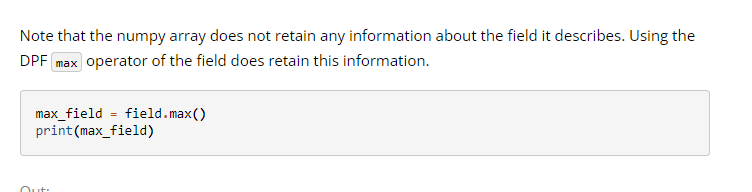# Find the maximum value in a field AND corresponding scoping IDMember, Employee Posts: 11edited October 24

I need to find the node with the max stress. How can I do this using DPF

Tagged:

•Member, Employee Posts: 169the end there is are a few lines to show how to get max and Ids of the field.•Member, Employee Posts: 169Example how to do this in the mechanical scripting window with dpf. The example is von mises stress, but you can change that line to any result.

```import mech_dpf
import Ans.DataProcessing as dpf

#Result Data
analysis1 = ExtAPI.DataModel.Project.Model.Analyses
dataSource = dpf.DataSources(analysis1.Solution.ResultFilePath)
#model
my_model = dpf.Model(analysis1.Solution.ResultFilePath)
#my mesh
my_mesh = my_model.Mesh

#time scoping
my_time_scoping = 1

#Time list

result_fc = dpf.operators.result.stress_von_mises(time_scoping=my_time_scoping,data_sources=dataSource).outputs.fields_container.GetData()
result_field = result_fc
max_min_op = dpf.operators.min_max.min_max(field=result_field)
max_id = max_min_op.outputs.field_max.GetData().ScopingIds
max_value = max_min_op.outputs.field_max.GetData().Data

min_id = max_min_op.outputs.field_min.GetData().ScopingIds
min_value = max_min_op.outputs.field_min.GetData().Data

max_result = [max_id,max_value]
min_result = [min_id,min_value]
print(max_result)
print(min_result)
```